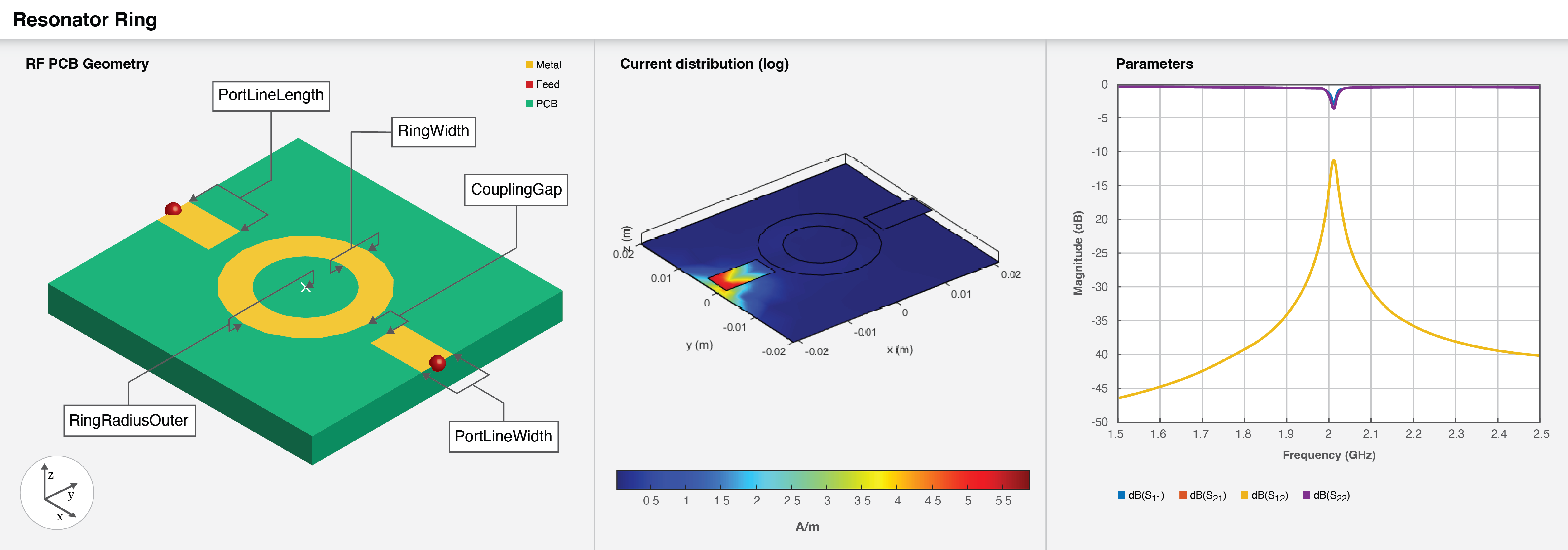# resonatorRing

Create gap-coupled ring resonator in microstrip form

## Description

Use the `resonatorRing` object to create a gap-coupled ring resonator in the microstrip form. Resonant structures such as rectangular, circular, and ring resonators are used in oscillators and filters.## Creation

### Syntax

``resonator = resonatorRing``
``resonator = resonatorRing(Name=Value)``

### Description

example

````resonator = resonatorRing` creates a default gap-coupled ring resonator. The default properties of the ring resonator are for a design frequency of 1 GHz.```

example

````resonator = resonatorRing(Name=Value)` sets Properties using one or more name-value arguments. For example, `resonatorRing(RingWidth=0.045)` creates a ring resonator with a ring width of `0.045` meters. Properties not specified retain their default values```

## Properties

expand all

Length of the port line in meters, specified as a positive scalar.

Example: ```resonator = resonatorRing(PortLineLength=0.045)```

Data Types: `double`

Width of the port line in meters, specified as a positive scalar.

Example: ```resonator = resonatorRing(PortLineWidth=0.0061)```

Data Types: `double`

Gap between the port lines and the ring in meters, specified as a positive scalar.

Example: ```resonator = resonatorRing(CouplingGap=0.002)```

Data Types: `double`

Outer radius of the ring in meters, specified as a positive scalar.

Example: ```resonator = resonatorRing(RingRadiusOuter=0.0481)```

Data Types: `double`

Width of the ring in meters, specified as a positive scalar.

Example: ```resonator = resonatorRing(RingWidth=0.0061)```

Data Types: `double`

Height of the resonator from the ground plane in meters, specified as a positive scalar. In the case of a multilayer substrate, you can use the `Height` property to create a resonator where the two dielectrics interface.

Example: `resonator = resonatorRing(Height=0.020)`

Data Types: `double`

Width of the ground plane in meters, specified as a positive scalar.

Example: ```resonator = resonatorRing(GroundPlaneWidth=0.2241)```

Data Types: `double`

Type of dielectric material used as a substrate, specified as a `dielectric` object. The thickness of the default dielectric material Teflon is `0.0016` m or the same value as the `Height` property.

Example: `d = dielectric("FR4");` `resonator = resonatorRing(Substrate=d)`

Type of metal used in the conducting layers, specified as a `metal` object.

Example: `m = metal("Copper");` `resonator = resonatorRing(Conductor=m)`

## Object Functions

 `charge` Calculate and plot charge distribution `current` Calculate and plot current distribution `design` Design ring resonator around specified frequency `feedCurrent` Calculate current at feed port `getZ0` Calculate characteristic impedance of transmission line `layout` Plot all metal layers and board shape `mesh` Change and view mesh properties of metal or dielectric in PCB component `shapes` Extract all metal layer shapes of PCB component `show` Display PCB component structure or PCB shape `sparameters` Calculate S-parameters for RF PCB objects

## Examples

collapse all

Create a default ring resonator.

`resonator = resonatorRing`
```resonator = resonatorRing with properties: PortLineLength: 0.0100 PortLineWidth: 0.0050 CouplingGap: 1.0000e-03 RingRadiusOuter: 0.0100 RingWidth: 0.0040 Height: 0.0016 GroundPlaneWidth: 0.0400 Substrate: [1x1 dielectric] Conductor: [1x1 metal] ```

Visualize the resonator.

`show(resonator)`Create a ring resonator at the interface of a multilayer dielectric.

```resonator = resonatorRing; resonator.Substrate = dielectric(Name={'Teflon','Teflon'},EpsilonR=[2.1 2.1],... LossTangent=[0 0],Thickness=[0.8e-3 0.8e-3]); resonator.Height = 0.8e-3;```

Visualize the ring resonator.

`show(resonator)`Pozar, David.M. Microwave Engineering Singapore; JohnWiley and Sons. Inc, 2012.

 Bogner, Andreas, Carsten Steiner, Stefanie Walter, Jaroslaw Kita, Gunter Hagen, and Ralf Moos. Planar Microstrip ring resonators for Microwave-Based Gas Sensing: Design Aspects and Initial Transducers for Humidity and Ammonia Sensing.

 Bernard, P.A., and J.M. Gautray. “Measurement of Dielectric Constant Using a Microstrip Ring Resonator.” IEEE Transactions on Microwave Theory and Techniques 39, no. 3 (March 1991): 592–95.